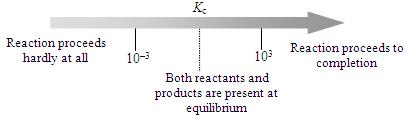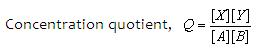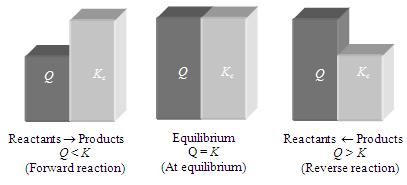1800-1023-196

+91-120-4616500

# Applications of Equilibrium Constant

## Applications of Equilibrium Constant

(1) Judging the extent of reaction

(i) If Kc > 103, products predominate over reactants. If Kc is very large, the reaction proceeds almost all the way to completion.

(ii) If Kc > 103, reactants predominate over products. If Kc is very small, the reaction proceeds hardly at all.

(iii) If Kc is in the range 103 to 103, appreaciable concentration of both reactants and products are present.(2) Predicting the direction of reaction: The concentration ratio, i.e., ratio of the product of concentrations of products to that of reactants is also known as concentration quotient and is denoted by Q.It may be noted that Q becomes equal to equilibrium constant (K) when the reaction is at the equilibrium state. At equilibrium, Q K = Kc = KpThus,

(i) If Q > K, the reaction will proceed in the direction of reactants (reverse reaction).

(ii) If Q < K, the reaction will proceed in the direction of the products (forward reaction).

(iii) If Q = K, the reaction mixture is already at equilibrium.

Thus, a reaction has a tendency to form products if Q < K and to form reactants if Q > K.## NEET & AIIMS Exam Sample Papers

 AIIMS SAMPLE PAPERS View More NEET SAMPLE PAPERS View More